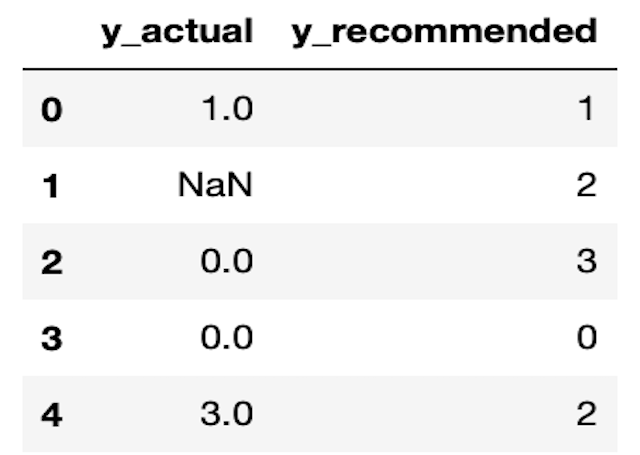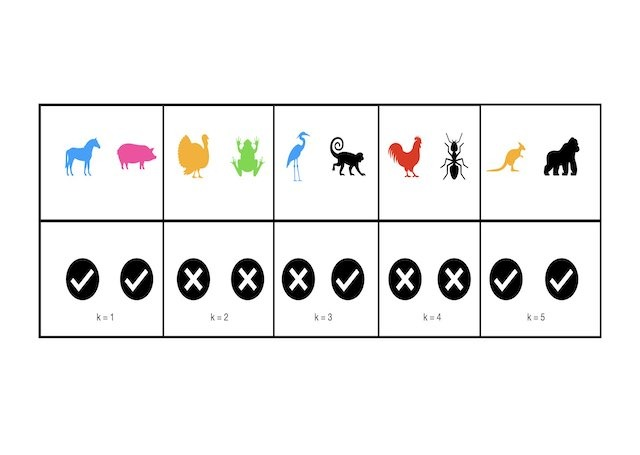# Supervised Models

This category groups articles dealing with supervised problems. Each post focuses on either a specific supervised algorithm, or a tool used when tackling a supervised problem. The emphasis here is on understanding these models and techniques at a technical level. Here you will learn to build supervised models in Python from scratch.## Are Decision Trees Robust to Outliers?

Are Decision Trees Robust to Outliers? In general, Decision Trees are quite robust to the presence of outliers in the data. This is true for both training and prediction. However, care needs to be taken to ensure the Decision Tree has been adequately regularised. An overfitted Decision Tree will show sensitivity to outliers. Why are …## How to Interpret Decision Trees with 1 Simple Example

How to Interpret Decision Trees with 1 Simple Example We can interpret Decision Trees as a sequence of simple questions for our data, with yes/no answers. One starts at the root node, where the first question is asked. Based upon the answer, we navigate to one of two child nodes. Each child node asks an additional …## 3 Methods to Tune Hyperparameters in Decision Trees

3 Methods to Tune Hyperparameters in Decision Trees We can tune hyperparameters in Decision Trees by comparing models trained with different parameter configurations, on the same data. An optimal model can then be selected from the various different attempts, using any relevant metrics. There are several different techniques for accomplishing this task. Three of the …## How to Measure Information Gain in Decision Trees

How to Measure Information Gain in Decision Trees For classification problems, information gain in Decision Trees is measured using the Shannon Entropy. The amount of entropy can be calculated for any given node in the tree, along with its two child nodes. The difference between the amount of entropy in the parent node, and the …## Precision@k and Recall@k Made Easy with 1 Python Example

Understanding the Adaboost Regression Algorithm For those who prefer a video presentation, you can see me work through the material in this post here: https://youtu.be/WEJcETfWwOo What are Precision@k and Recall@K ? Precision@k and Recall@k are metrics used to evaluate a recommender model. These quantities attempt to measure how effective a recommender is at providing relevant suggestions …## Implement the KNN Algorithm in Python from Scratch

Implement the KNN Algorithm in Python from Scratch In this post, we will cover the K Nearest Neighbours algorithm: how it works and how it can be used. We will work through implementing this algorithm in Python from scratch, and verify that our model works as expected. What is the KNN Algorithm? K Nearest Neighbours …## Implement Gradient Boosting Regression in Python from Scratch

Implement Gradient Boosting Regression in Python from Scratch In this post, we will implement the Gradient Boosting Regression algorithm in Python. This is a powerful supervised machine learning model, and popularly used for prediction tasks. To gain a deep insight into how this algorithm works, the model will be built up from scratch, and subsequently verified against the …## Understanding the Gradient Boosting Regressor Algorithm

Understanding the Gradient Boosting Regressor Algorithm In this post, we will cover the Gradient Boosting Regressor algorithm: the motivation, foundational assumptions, and derivation of this modelling approach. Gradient boosters are powerful supervised algorithms, and popularly used for predictive tasks. Motivation: Why Gradient Boosting Regressors? The Gradient Boosting Regressor is another variant of the boosting ensemble technique that …## A Complete Introduction to Cross Validation in Machine Learning

A Complete Introduction to Cross Validation in Machine Learning This post will discuss various Cross Validation techniques. Cross Validation is a testing methodology used to quantify how well a predictive machine learning model performs. Simple illustrative examples will be used, along with coding examples in Python. What is Cross Validation? A natural question to ask, when …